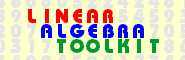© 2000−2019  P. Bogacki Linear Algebra Toolkit - Main Page v. 1.25

This Linear Algebra Toolkit is composed of the modules listed below. Each module is designed to help a linear algebra student learn and practice a basic linear algebra procedure, such as Gauss-Jordan reduction, calculating the determinant, or checking for linear independence.

 MODULES

 Systems of linear equations and matrices Row operation calculator Interactively perform a sequence of elementary row operations on the given m x n matrix A. Transforming a matrix to row echelon form Find a matrix in row echelon form that is row equivalent to the given m x n matrix A. Transforming a matrix to reduced row echelon form Find the matrix in reduced row echelon form that is row equivalent to the given m x n matrix A. Solving a system of linear equations Solve the given system of m linear equations in n unknowns. Calculating the inverse using row operations Find (if possible) the inverse of the given n x n matrix A. Determinants Calculating the determinant using row operations Calculate the determinant of the given n x n matrix A. Vector spaces Linear independence and dependence Given the set S = {v1, v2, ... , vn} of vectors in the vector space V, determine whether S is linearly independent or linearly dependent. Determining if the set spans the space Given the set S = {v1, v2, ... , vn} of vectors in the vector space V, determine whether S spans V. Finding a basis of the space spanned by the set Given the set S = {v1, v2, ... , vn} of vectors in the vector space V, find a basis for span S. Finding a basis of the null space of a matrix Find a basis of the null space of the given m x n matrix A. (Also discussed: rank and nullity of A.) Linear transformations Finding the kernel of the linear transformation Find the kernel of the linear transformation L: V→W. (Also discussed: nullity of L; is L one-to-one?) Finding the range of the linear transformation Find the range of the linear transformation L: V→W. (Also discussed: rank of L; is L onto W?)

• The Linear Algebra Toolkit has been written entirely in PERL. Every effort has been made to make it compatible with a broad range of browsers, however, no guarantee can be made that every browser will properly handle this application.

• In order to bookmark the toolkit, first go to the cover page; all the other pages in this toolkit (including the one you are reading) are created dynamically.

• The toolkit represents all the data (such as matrix entries, vector components and polynomial coefficients) as rational numbers, where both the numerator and denominator are stored as integers. All operations on these rational numbers are performed exactly. Note that in the current version overflow errors are not trapped.

• Permission is hereby granted for any noncommercial use of this product by individuals, e.g. students, faculty, etc.
Permission for the class use of this product in an academic environment is automatically granted, provided the instructor notifies the author.
Any other use (including any commercial use) requires an explicit permission from the author.

• The textbook Linear Algebra: Concepts and Applications published in 2019 by MAA Press (an imprint of the American Mathematical Society) contains numerous references to the Linear Algebra Toolkit.
This book is available at Google Play and Amazon.

• Comments should be forwarded to the author:

Przemyslaw Bogacki.
Department of Mathematics and Statistics
Old Dominion University
Norfolk, VA 23529

Phone: (757) 683-3262
E-mail: pbogacki@odu.edu

You can read testimonials from students and instructors who used the Toolkit.

• These pages should be considered work in progress. The interface and functionality will undergo changes as new versions are released.
Here is a list of all released versions:
VersionDate releasedDescription
1.00May 6, 2000Row Operation Calculator
1.20September 6, 2000ROC becomes Linear Algebra Toolkit